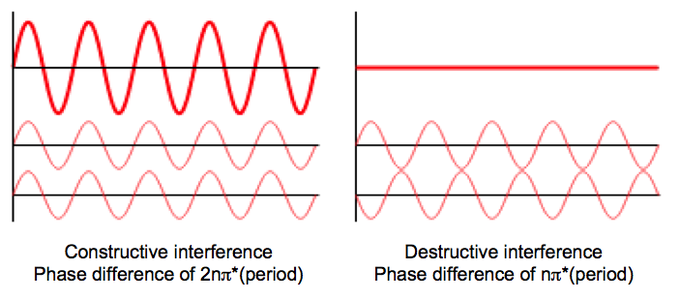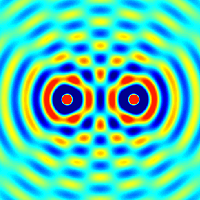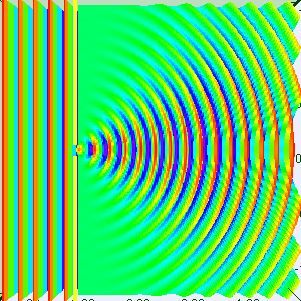## Interference and Diffraction

#### Learning Objective

• Recognize the difference between constructive and destructive interference, and between interference and diffraction

#### Key Points

• In physics, interference is a phenomenon in which two waves superimpose to form a resultant wave of greater or lower amplitude.
• Constructive interference occurs when the phase difference between the waves is a multiple of 2π, whereas destructive interference occurs when the difference is π, 3π, 5π, etc.
• Diffraction refers to various phenomena that occur when a wave encounters an obstacle. In classical physics, the diffraction phenomenon is described as the apparent bending of waves around small obstacles and the spreading out of waves past small openings.

#### Terms

• interferenceAn effect caused by the superposition of two systems of waves, such as a distortion on a broadcast signal due to atmospheric or other effects. In physics, interference is a phenomenon in which two waves superimpose to form a resultant wave of greater or lower amplitude.
• amplitudeThe maximum absolute value of some quantity that varies, especially a wave.
• diffractionThe breaking up of an electromagnetic wave as it passes a geometric structure (e.g., a slit), followed by reconstruction of the wave by interference.

In physics, interference is a phenomenon in which two waves superimpose to form a resultant wave of greater or lower amplitude. Interference usually refers to the interaction of waves that are correlated or coherent with each other, either because they come from the same source or because they have the same (or nearly the same) frequency. Interference effects can be observed with all types of waves, including light, radio, acoustic, and surface water waves. In chemistry, the applications of interference to light are the most relevant to the study of matter.

## Mechanism of Interference

The principle of superposition of waves states that when two or more waves are incident on the same point, the total displacement at that point is equal to the vector sum of the displacements of the individual waves. If a crest of a wave meets a crest of another wave of the same frequency at the same point, then the magnitude of the displacement is the sum of the individual magnitudes; this is known as constructive interference. If a crest of one wave meets a trough of another wave, then the magnitude of the displacements is equal to the difference in the individual magnitudes; this is known as destructive interference.Interference of two wavesThese two examples represent constructive (left) and destructive interference (right) in wave phenomena. When the two waves are “in phase,” their periods are offset by 2nπ*period. However, when they are precisely out of phase, destructive interference results if the phase difference is nπ*period.

Constructive interference occurs when the phase difference between the waves is a multiple of 2π, whereas destructive interference occurs when the difference is π, 3π, 5π, etc. If the difference between the phases is intermediate between these two extremes, then the magnitude of the displacement of the summed waves lies between the minimum and maximum values.

Consider, for example, what happens when two identical stones are dropped into a still pool of water at different locations. Each stone generates a circular wave propagating outwards from the point where the stone was dropped. When the two waves overlap, the net displacement at a particular point is the sum of the displacements of the individual waves. At some points, these will be in phase and will produce a maximum displacement. In other places, the waves will be in anti-phase and there will be no net displacement at these points. Thus, parts of the surface will be stationary.Two Sources of InterferenceThe effect of two waves interfering with each other, for example, two stones thrown into a pool of water.

## Diffraction

Diffraction refers to various phenomena that occur when a wave encounters an obstacle. In classical physics, the diffraction phenomenon is described as the apparent bending of waves around small obstacles and the spreading out of waves past small openings. Similar effects occur when light waves travel through a medium with a varying refractive index or a sound wave through one with varying acoustic impedance. Diffraction occurs with all waves, including sound waves, water waves, and electromagnetic waves such as visible light, X-rays, and radio waves. As physical objects have wave-like properties (at the atomic level), diffraction also occurs with matter and can be studied according to the principles of quantum mechanics. Italian scientist Francesco Maria Grimaldi coined the word diffraction and was the first to record accurate observations of the phenomenon in 1665.DiffractionIn classical physics, the diffraction phenomenon is described as the apparent bending of waves around small obstacles and the spreading out of waves past small openings.

The effects of diffraction are often seen in everyday life. The most striking examples of diffraction are those involving light; for example, the closely spaced tracks on a CD or DVD act as a diffraction grating to form the familiar rainbow pattern seen when looking at a disk. This principle can be extended to engineer a grating with a structure such that it will produce any diffraction pattern desired; the hologram on a credit card is an example. Diffraction in the atmosphere by small particles can cause a bright ring to be visible around a bright light source like the sun or the moon. A shadow of a solid object, using light from a compact source, shows small fringes near its edges. All these effects occur because light propagates as a wave.

Richard Feynman said, “No one has ever been able to define the difference between interference and diffraction satisfactorily. It is just a question of usage, and there is no specific, important physical difference between them.”

He suggested that when there are only a few sources, say two, we call it interference (as in Young’s slits), but with a large number of sources, the process can be labelled diffraction.

While diffraction occurs whenever propagating waves encounter such changes, its effects are generally most pronounced for waves where the wavelength is roughly similar to the dimensions of the diffracting objects. If the obstructing object provides multiple, closely spaced openings, a complex pattern of varying intensity can result. This is due to the superposition, or interference, of different parts of a wave that traveled to the observer by different paths (see diffraction grating).